Generic filters
Exact matches only

# How to Get Sloths Out of Your Steiner TreeBack in school, a professor put on a demonstration that has stuck with me over the years. He put two computers side by side: one a 12-year-old brick of a laptop and the other a brand new MacBook. Both were tasked with the same set of sorting problems. With the smaller problems, the race was close; but as they reached the larger sorting problems, the 12-year-old laptop started pulling ahead.

## The Steiner Tree Algorithm

How was it possible for a state-of-the-art machine to lose to something that functions better as a doorstop than as a computer? Turns out, the difference was algorithmic: one machine was running quicksort and the other bubble sort. It didn’t matter that the MacBook was 20 times more powerful than the other computer because its code was slow. This was a formative part of my introduction to algorithms.

As a software engineer, I seldom get to work on algorithmic problems, which is a shame because algorithms are cool and interesting. The good ones, anyway. There is an elegance in knowing, mathematically, how fast a solution runs. Recently, though, a client issue gave me the chance to dive into a complex graph algorithm known as the Steiner Tree Problem, which on its surface looks deceptively simple: Given an undirected graph and a subset of nodes, the Steiner Tree Problem asks you to find the lowest weight subgraph that contains each node. Sounds straightforward, but it actually took weeks to turn this puzzle into a workable solution. This is the story of that solution, but first, let me provide a little more context on the client issue.

## The Challenge

In Exago BI, users can design reports using a tool called ExpressView, which allows them to build data models on the fly simply by dragging data fields onto a canvas. Exago BI will create the best join path based on the data framework and what is on the canvas at run time. The data framework approach was developed so users could create effective reports without knowing anything about joins or the underlying data model or being restricted to data models built for them in advance. That join path gets translated into SQL, so the better the join path, the better the database performance.

The client issue in question featured an ExpressView that would not execute. The report wasn’t anything spectacular either: there were no complex filters or intricate extensions, just two data fields from two different entities (a.k.a. data objects). Interestingly, it didn’t matter which two columns they were. As long as the fields were from different data objects, the execution hung. Some further digging revealed that the data model was unusually large. The algorithm had not previously had any trouble navigating smaller data frameworks but was struggling to process the multitude of entities in this client’s environment. The application wasn’t hitting the database because it was stuck calculating the join path. What would normally be a lightning-quick execution was moving at a sloth’s pace and with about as much urgency.

Of course, we could have just instructed the client to break their data framework up into multiple data models, thereby restricting users to a subset of the environment’s entities, but that would mean more overhead for the client and less freedom for their users. I saw this issue ticket as an opportunity to further optimize ExpressView and make it an even more powerful alternative to traditional reporting. So we decided to go the algorithmic route instead.

## Dijkstra’s: Finding the Shortest Path

The best way to tackle this problem was to represent the database as a graph, with the data entities expressed as nodes and the joins between them as edges. The entities on the client’s ExpressView are two nodes that I need to find the most efficient way to connect. It is a shortest path problem.

Generally, there are two camps that search algorithms fall into: informed and uninformed. Informed search algorithms are faster but use heuristic functions to “guess” at the next step to take. Uninformed algorithms are slower but have the benefit of being less specialized and therefore more widely applicable. An informed algorithm was out of the picture from the get-go because Exago BI deals with a multitude of databases (or in this case, graphs) and each one is unique. Creating a heuristic function that would work for all databases is almost impossible. This leaves us with uninformed search algorithms.

Of the uninformed search algorithms, I decided on Dijkstra’s. Dijkstra’s algorithm, created in 1956 by Edsger W. Dijkstra, is a shortest path algorithm that these days is commonly used in routing protocols and as part of other algorithms. I chose it because it performs well on sparse graphs and has a small memory footprint. Sparse graphs have few edges compared to the number of nodes. It’s safe to assume that if a client has a large environment, it is probably a sparse graph. In the unlikely event a client has a dense graph (wherein the number of edges is approaching the maximum number of edges possible), the graph will be small enough that difference will be imperceptible to the end user. Dijkstra’s algorithm, using a priority queue, has a run time of O(E + VLog(V)) where V is the number of nodes and E is the number of edges.

With regard to memory, each node just needs to hold a pointer to the next node in the shortest path. The path is retrieved by starting at the ending node and backtracking to the start. By holding the shortest path in the nodes of the graph, the memory complexity is the same as the graph representation of the database.

So there it is. Problem solved, right? Maybe take an early lunch and move onto some real problems.

Unfortunately, no. Dijkstra’s algorithm is great when a user is only working with two entities, but what if he or she needs three? Or four? Suddenly, the problem is more complicated. Depending on the graph and the input, calling Dijkstra’s for each extra node can result in the database getting passed more joins than expected.

Since what we need is the smallest subgraph that contains each of the entities on the report, what we’re really looking at is the Steiner tree problem.

## Is Steiner Tree an NP-Hard Problem?

The Steiner tree problem is classified as NP-Hard, meaning there is no known algorithm (and probably no possible algorithm) that can find the optimal solution under polynomial time. Popular optimal solutions to the Steiner tree problem run around O(3V). For reference, with an input size of 40, that is 10 times more operations than grains of sand on earth. A processor running at 300,000 million instructions per second would need over 469 days to compute it, so a sloth moving at an average speed of .15 mph could travel 4221 miles before the report executed.

## The Steiner Tree: The “Good Enough” Solution

Things aren’t as bad as they might seem. Generally, the solution to NP-Hard problems is to take the heuristic or “good enough” approach. It isn’t worth exploring an exponential search space for the exact solution if our end goal is a snappy and responsive designer. In the interest of moving faster than a sloth, we can make an approximate solution. While there might be a few extra joins in the output, we’ll get the result in seconds rather than years.

Here is the high level algorithm I used to solve the Steiner tree problem:

Starting with the graph below, our goal is to find the Steiner tree that includes the points v1, v2, v3, and v4.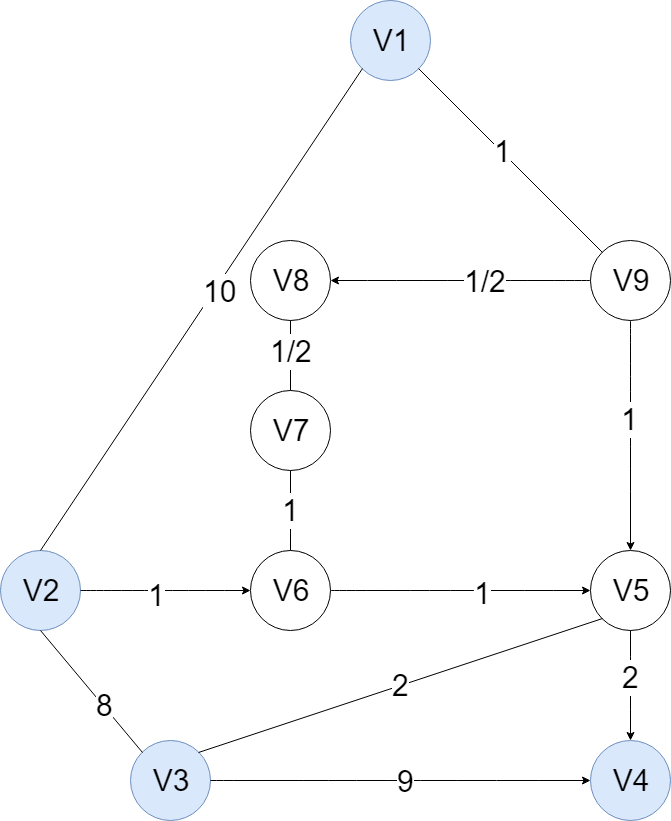1) Construct the complete distance graph (G). The first step is to create a complete graph that is made up of each Steiner point. Each edge is the cost of the shortest path between those two points. Even though there is a direct path between v1 and v2, for example, it is discarded in favor of a more circuitous but lower-cost path that includes nodes 6 – 9.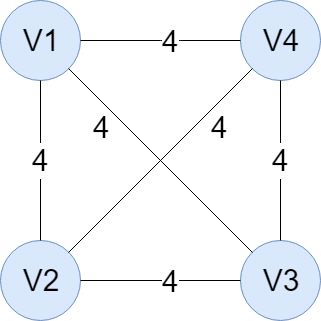2) Find the Minimum Spanning Tree (T) of G. Finding the MST of G gets us the lowest cost of all the shortest paths that still spans each Steiner point. In this example, there is more than one MST, so we just choose any arbitrary one.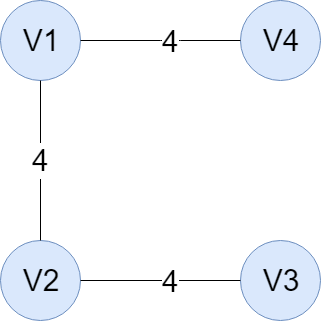3) Create a subgraph (G’) by replacing edges in T with the corresponding shortest path in G. For instance, the edge between v1 and v2 in graph T is replaced with the corresponding shortest path in G. Instead of those nodes being directly joined, we add v9, v8, v7, and v6 and the edges between them.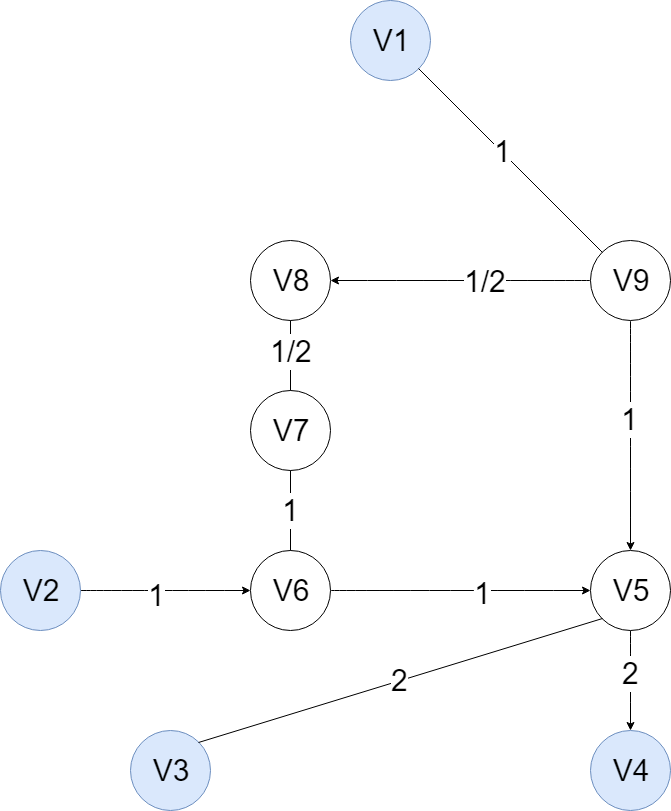4) Find the Minimum Spanning Tree (T’) of G’. By finding the MST after step 3, we can see exactly what pathways are redundant. Here, the path between v6 and v7 is removed because v7 is joined to the rest of the graph through v8 and v9.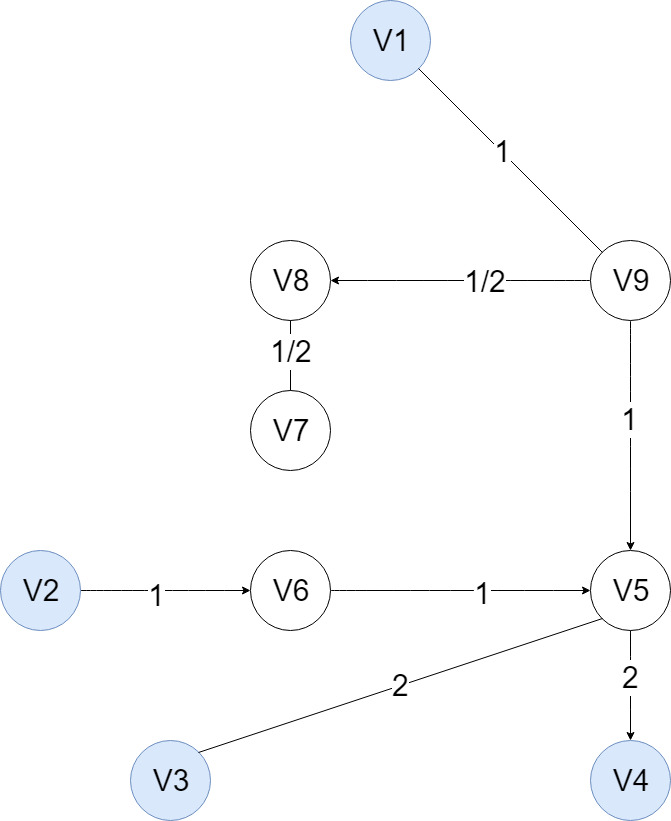5) Create a Steiner Tree (F) from T’ by deleting edges in T’ so all the terminal nodes in F are Steiner points. The last step is to remove any extra terminal nodes that are unnecessary (as in not one of the required nodes). What we’re left with is our final Steiner tree.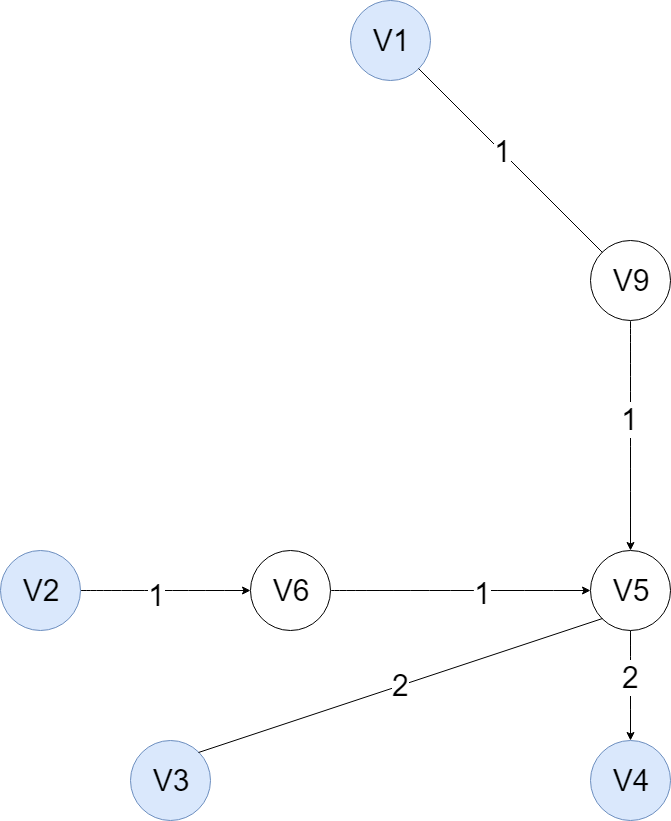Ok. So that’s the high level algorithm. But a high level algorithm isn’t the end of the road. Each step in the high level algorithm is an algorithm itself, and choosing the right one for the job is critical.

## The Floyd Warshall Algorithm

To find the complete distance graph for Step 1 in the high-level algorithm, the shortest distance between all nodes needs to be computed. This is also known as the all-pairs shortest path problem. In theory, Dijkstra’s implementation used in the shortest path problem could be reused here.

This, however, would cause a scaling issue. Dijkstra’s uses the nodes of the graph to store the shortest path, which works fine when run once. Now that the algorithm needs to be run multiple times, the nodes in the graph need to get reset each run, thereby clearing the previously stored shortest path. Extracting the path is possible but requires a new object to hold it. It’s inefficient, but not if we implement the Floyd Warshall algorithm. Floyd Warshall uses a V by V adjacency matrix to store the shortest path between any two nodes and has a run time O(V3). Compared to Dijkstra’s O(EV + V2log(V)) repeated runtime, it seems slow, and on sparse graphs it is. But the fact that every shortest path can be fit into an elegant data structure is a huge plus.

## Analysis Paralysis

Now we have a complete graph comprised of each Steiner points and the shortest path between them. The next step is to find the minimum spanning tree of said graph. The two algorithms I considered here were Kruskal’s and Prim’s algorithms. Between the two, Kruskal’s algorithm has a better run time for sparse graphs, making it the obvious choice. However, the input for Step 2 in the process outlined above is always a complete graph and therefore a dense graph. Prim’s algorithm is problematic due to our inability to predict graph density when the algorithm is run a second time (Step 4). So how to choose?

In this situation, I was forced to simply pick one. It’s impossible to predict the form of the graph before Step 4 since it is dependent on user input. All that is known is that it is possible to join the entities from the input.

This is a classic case of analysis paralysis. I can spend all day thinking about graphs and the shortest paths, but is this productive? Does it get me closer to a working solution? At the end of the day, Steps 2 and 4 are going to take a miniscule amount of time compared to running Floyd Warshall. The best algorithm is the one that gets me moving. I ended up implementing Kruskal’s Algorithm, which has a run time of run time of O(E log(E)), because it was the easier of the two to implement. Keep it simple, stupid.

## The Run Time

Algorithmically, Steps 3 and 5 are unremarkable. Replacing all of the edges with the shortest path between Steiner points (Step 3) can be accomplished in O(E). Removing any terminals that are not steiner points (Step 5) is done in O(V2). So our total run time is O(V3 + 2 (E log(E)) + E + V2) or simply O(V3) in the worst case. With an input size of 40 nodes, the approximation algorithm will take about .0000000213 seconds. I.e., the sloth hasn’t even thought about moving yet.

How about the space complexity? As previously stated, Floyd Warshall uses a V by V matrix where it takes O(V2) to find the All-pairs shortest paths. Kruskal’s algorithm works by removing edges and nodes from the graph provided, so finding the minimum spanning tree doesn’t need any extra memory. Replacing edges and removing loosed terminal nodes morph the graph itself.

And that’s it. The total space requirement to run this implementation is a O(V2) adjacency matrix. That’s pretty cool.

For SaaS businesses looking for embedded BI solutions, Exago supports providers and their users by making it easy for business professionals to access and manipulate their data, no matter their technical qualifications. Exago BI integrates seamlessly with web-based SaaS applications to bring ad hoc reporting and analytics to any multi-tenant environment. Our dedication to customizable integration allows us to prioritize usability without compromising on power. Contact us today for more information.Stay up-to-date on all things SaaS and analytics with fresh content each month.